niuchao

• 1

获得赞
• 1

发布的文章
• 0

答辩的项目

## 深度学习中的过拟合、欠拟合及其解决方案研究

K折交叉验证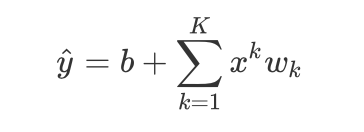来近似 。在上式中，wk是模型的权重参数，是偏差参数。与线性回归相同，多项式函数拟合也使用平方损失函数。特别地，一阶多项式函数拟合又叫线性函数拟合。 给定训练数据集，模型复杂度和误差之间的关系：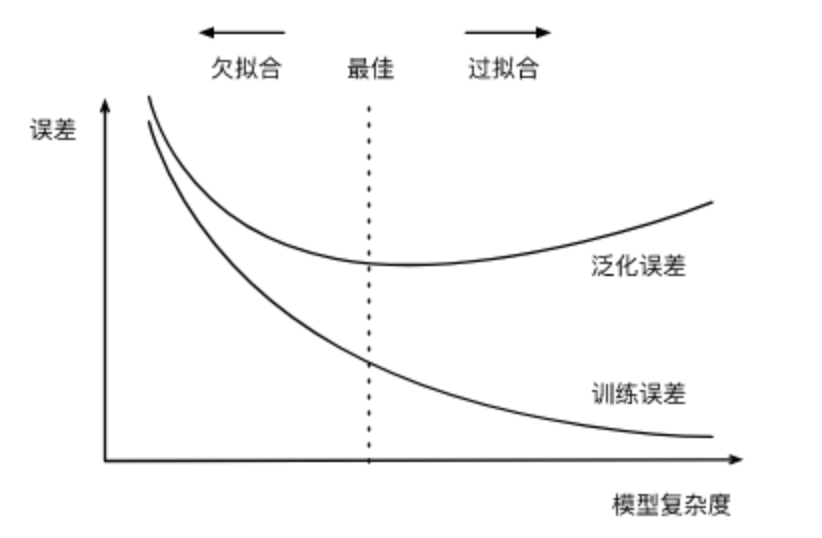训练数据集大小

```%matplotlib inline
import torch
import numpy as np
import sys
sys.path.append("/home/kesci/input")
import d2lzh1981 as d2l
print(torch.__version__)```

```n_train, n_test, true_w, true_b = 100, 100, [1.2, -3.4, 5.6], 5
features = torch.randn((n_train + n_test, 1))
poly_features = torch.cat((features, torch.pow(features, 2), torch.pow(features, 3)), 1)
labels = (true_w * poly_features[:, 0] + true_w * poly_features[:, 1]
+ true_w * poly_features[:, 2] + true_b)
labels += torch.tensor(np.random.normal(0, 0.01, size=labels.size()), dtype=torch.float)```
`features[:2], poly_features[:2], labels[:2]`

```(tensor([[-0.8589],
[-0.2534]]), tensor([[-0.8589,  0.7377, -0.6335],
[-0.2534,  0.0642, -0.0163]]), tensor([-2.0794,  4.4039]))```

```def semilogy(x_vals, y_vals, x_label, y_label, x2_vals=None, y2_vals=None,
legend=None, figsize=(3.5, 2.5)):
# d2l.set_figsize(figsize)
d2l.plt.xlabel(x_label)
d2l.plt.ylabel(y_label)
d2l.plt.semilogy(x_vals, y_vals)
if x2_vals and y2_vals:
d2l.plt.semilogy(x2_vals, y2_vals, linestyle=':')
d2l.plt.legend(legend)```
```num_epochs, loss = 100, torch.nn.MSELoss()

def fit_and_plot(train_features, test_features, train_labels, test_labels):
# 初始化网络模型
net = torch.nn.Linear(train_features.shape[-1], 1)
# 通过Linear文档可知，pytorch已经将参数初始化了，所以我们这里就不手动初始化了

# 设置批量大小
batch_size = min(10, train_labels.shape)
dataset = torch.utils.data.TensorDataset(train_features, train_labels)      # 设置数据集
train_iter = torch.utils.data.DataLoader(dataset, batch_size, shuffle=True) # 设置获取数据方式

optimizer = torch.optim.SGD(net.parameters(), lr=0.01)                      # 设置优化函数，使用的是随机梯度下降优化
train_ls, test_ls = [], []
for _ in range(num_epochs):
for X, y in train_iter:                                                 # 取一个批量的数据
l = loss(net(X), y.view(-1, 1))                                     # 输入到网络中计算输出，并和标签比较求得损失函数
l.backward()                                                        # 求梯度
optimizer.step()                                                    # 迭代优化函数，进行参数优化
train_labels = train_labels.view(-1, 1)
test_labels = test_labels.view(-1, 1)
train_ls.append(loss(net(train_features), train_labels).item())         # 将训练损失保存到train_ls中
test_ls.append(loss(net(test_features), test_labels).item())            # 将测试损失保存到test_ls中
print('final epoch: train loss', train_ls[-1], 'test loss', test_ls[-1])
semilogy(range(1, num_epochs + 1), train_ls, 'epochs', 'loss',
range(1, num_epochs + 1), test_ls, ['train', 'test'])
print('weight:', net.weight.data,
'\nbias:', net.bias.data)```

`fit_and_plot(poly_features[:n_train, :], poly_features[n_train:, :], labels[:n_train], labels[n_train:])`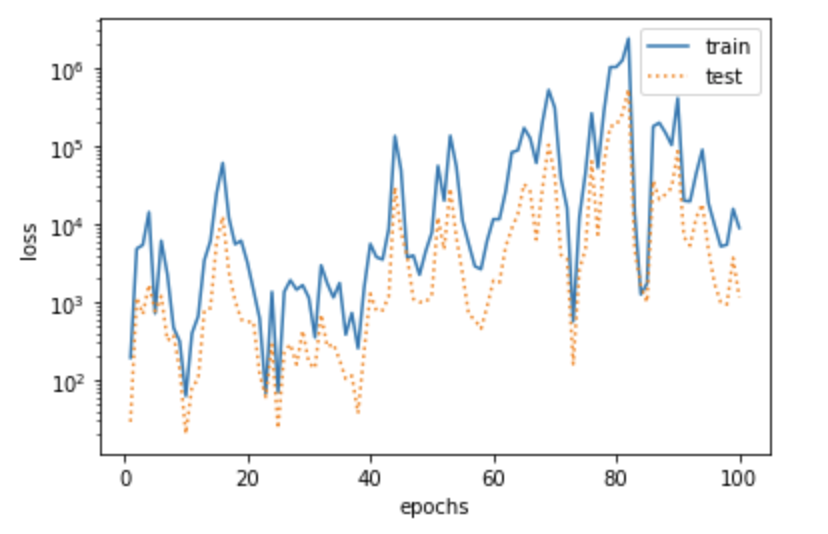`fit_and_plot(features[:n_train, :], features[n_train:, :], labels[:n_train], labels[n_train:])`
```final epoch: train loss 781.689453125 test loss 329.79852294921875
weight: tensor([[26.8753]])
bias: tensor([6.1426])```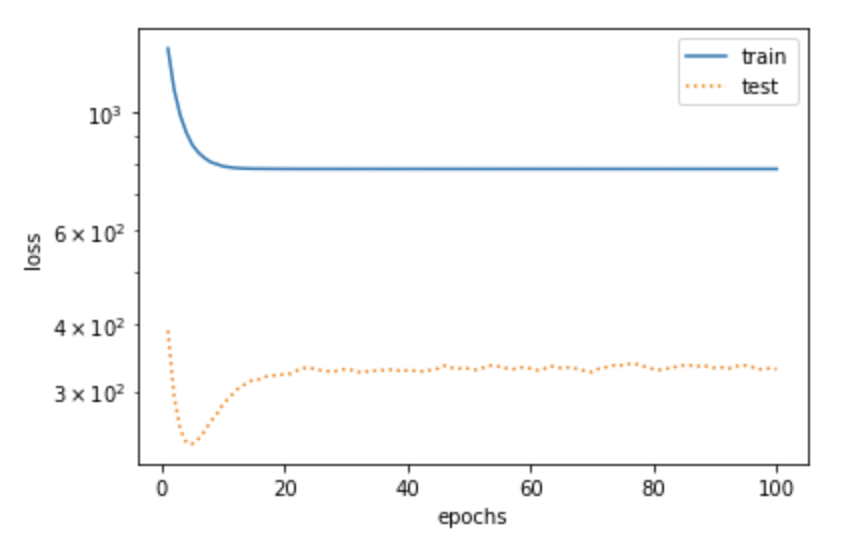`fit_and_plot(poly_features[0:2, :], poly_features[n_train:, :], labels[0:2], la`
```final epoch: train loss 6.23520565032959 test loss 409.9844665527344
weight: tensor([[ 0.9729, -0.9612,  0.7259]])
bias: tensor([1.6334])```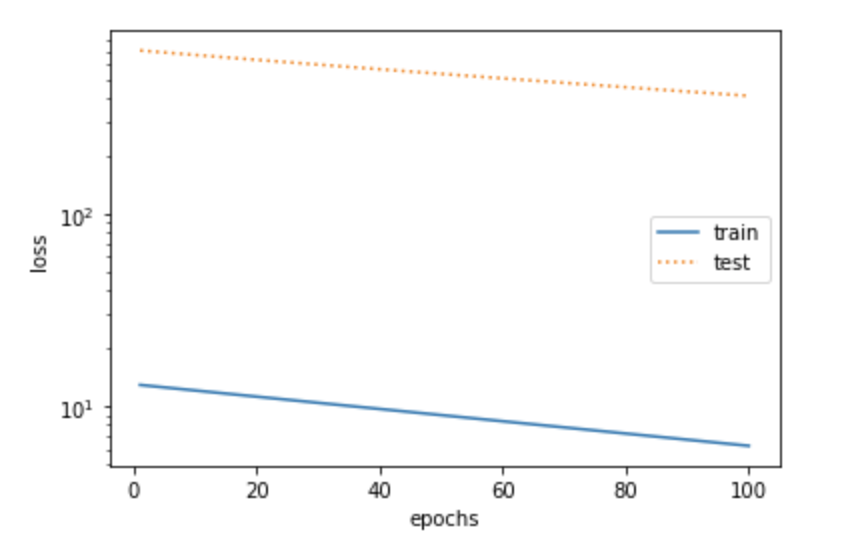权重衰减

L2 范数正则化（regularization）

L2范数正则化在模型原损失函数基础上添加范数惩罚项，从而得到训练所需要最小化的函数。L2范数惩罚项指的是模型权重参数每个元素的平方和与一个正的常数的乘积。以线性回归中的线性回归损失函数为例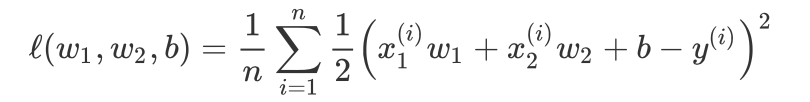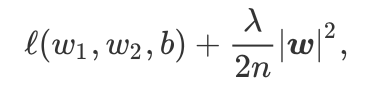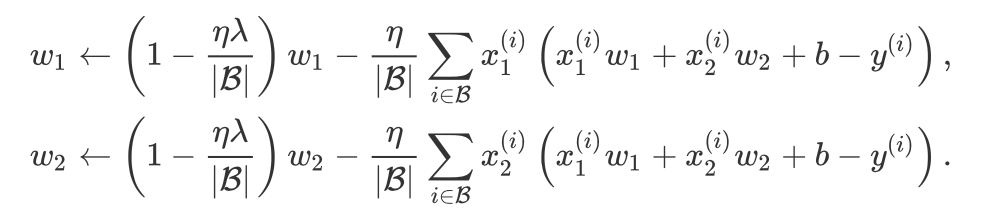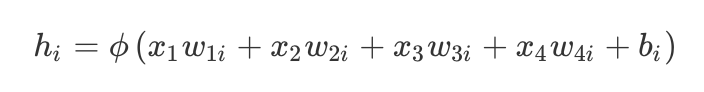这里激活函数，x1-x4是输入，隐藏单元i的权重参数为w1i,...,w4i，偏差参数为bi。当对该隐藏层使用丢弃法时，该层的隐藏单元将有一定概率被丢弃掉。设丢弃概率为p，那么有p的概率hi会被清零，有1-p的概率hi会除以1-p做拉伸。丢弃概率是丢弃法的超参数。具体来说，设随机变量为0和1的概率分别p为和1-p。使用丢弃法时我们计算新的隐藏单元hi'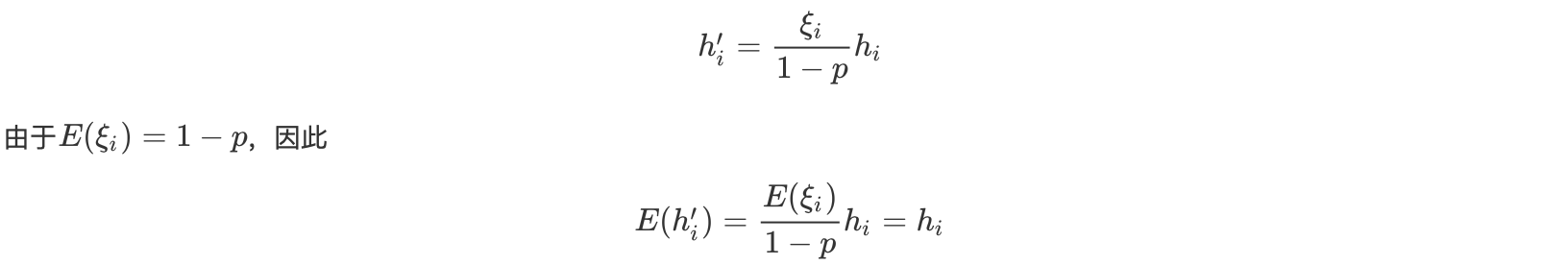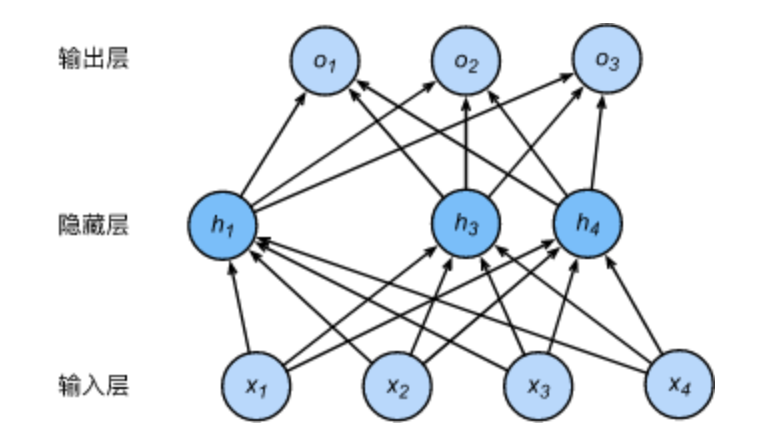500字niuchao

• 1

获得赞
• 1

发布的文章
• 0

答辩的项目